# Scale-space theory

(diff) ← Older revision | Latest revision (diff) | Newer revision → (diff)

A theory of multi-scale representation of sensory data developed by the image processing and computer vision communities. The purpose is to represent signals at multiple scales in such a way that fine scale structures are successively suppressed, and a scale parameteris associated with each level in the multi-scale representation.

For a given signal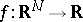, a linear scale-space representation is a family of derived signals, defined by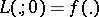and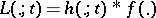for some family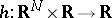of convolution kernels [a1], [a2] (cf. also Integral equation of convolution type). An essential requirement on the scale-space familyis that the representation at a coarse scale constitutes a simplification of the representations at finer scales. Several different ways of formalizing this requirement about non-creation of new structures with increasing scales show that the Gaussian kernel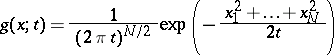constitutes a canonical choice for generating a scale-space representation [a3], [a4], [a5], [a6]. Equivalently, the scale-space family satisfies the diffusion equationThe motivation for generating a scale-space representation of a given data set originates from the basic fact that real-world objects are composed of different structures at different scales and may appear in different ways depending on the scale of observation. For example, the concept of a "tree" is appropriate at the scale of meters, while concepts such as leaves and molecules are more appropriate at finer scales. For a machine vision system analyzing an unknown scene, there is no way to know what scales are appropriate for describing the data. Thus, the only reasonable approach is to consider descriptions at all scales simultaneously [a1], [a2].

From the scale-space representation, at any level of scale one can define scale-space derivatives by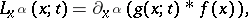where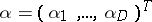and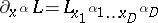constitute multi-index notation for the derivative operator. Such Gaussian derivative operators provide a compact way to characterize the local image structure around a certain image point at any scale. Specifically, the output from scale-space derivatives can be combined into multi-scale differential invariants, to serve as feature detectors (see Edge detection and Corner detection for two examples).

More generally, a scale-space representation with its Gaussian derivative operators can serve as a basis for expressing a large number of early visual operations, including feature detection, stereo matching, computation of motion descriptors and the computation of cues to surface shape [a3], [a4]. Neuro-physiological studies have shown that there are receptive field profiles in the mammalian retina and visual cortex, which can be well modeled by the scale-space framework [a7].

Pyramid representation [a8] is a predecessor to scale-space representation, constructed by simultaneously smoothing and subsampling a given signal. In this way, computationally highly efficient algorithms can be obtained. A problem noted with pyramid representations, however, is that it is usually algorithmically hard to relate structures at different scales, due to the discrete nature of the scale levels. In a scale-space representation, the existence of a continuous scale parameter makes it conceptually much easier to express this deep structure [a2]. For features defined as zero-crossings of differential invariants, the implicit function theorem (cf. Implicit function) directly defines trajectories across scales, and at those scales where a bifurcation occurs, the local behaviour can be modeled by singularity theory [a3], [a5].

Extensions of linear scale-space theory concern the formulation of non-linear scale-space concepts more committed to specific purposes [a9]. There are strong relations between scale-space theory and wavelet theory (cf. also Wavelet analysis), although these two notions of multi-scale representation have been developed from slightly different premises.

How to Cite This Entry:
Scale-space theory. Encyclopedia of Mathematics. URL: http://encyclopediaofmath.org/index.php?title=Scale-space_theory&oldid=11727
This article was adapted from an original article by Tony Lindeberg (originator), which appeared in Encyclopedia of Mathematics - ISBN 1402006098. See original article